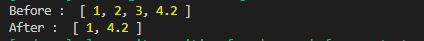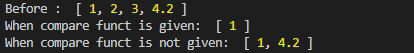Open in App
Not now

# Lodash _.differenceBy() Method

• Last Updated : 25 Mar, 2022

The _.differenceBy() method is used to remove the values from the original array by iterating over each element in the array by using the Iterate function. It is almost same as _.difference() function.

Syntax:

`lodash.differenceBy(array, [values], [iterate=_.identity])`

Parameters: This function accepts three parameters as mention above and describe below

• array: This is the array from which the values are to be removed.
• values: It is the Array of values that is to be removed from the original array.
• Iterate: This is the function that iterate over each element.

Note: If the iterate function is not given then _.differenceBy() function act as _.difference() function.

Return Value: This function returns an array.

Example 1:

## Javascript

 `// Requiring the lodash library` `const _ = require(``"lodash"``);`   `// Original array` `let array1 = [1, 2, 3, 4.2]`   `// Array to be subtracted` `let val = [2, 3, 3, 5]`   `// New Array after _.differenceBy() ` `// method where Math.double is the` `// comparable function` `let newArray = _.differenceBy(` `    ``array1, val, Math.double);`   `// Printing the original array` `console.log(``"Before : "``, array1);`   `// Printing the output` `console.log(``"After : "``, newArray);`

Output:Example 2:

## Javascript

 `// Requiring the lodash library` `const _ = require(``"lodash"``);`   `// Original array` `let array1 = [1, 2, 3, 4.2]`   `// Value array to be subtracted` `let val = [2, 3, 4, 5]`   `// new Array after _.differenceBy()` `// method where Math.double is the` `// comparable function` `let newArray1 = _.differenceBy(` `    ``array1, val, Math.floor);`   `// New Array after _.differenceBy function` `// where no comparable function is given` `let newArray2 = _.differenceBy(array1, val);`   `// Printing the original array` `console.log(``"Before : "``, array1);`   `// printing the output` `console.log(``"When compare funct is given: "``, ` `        ``newArray1);`   `// Printing the output` `console.log(``"When compare funct is not given: "``, ` `        ``newArray2);`

Output:My Personal Notes arrow_drop_up
Related Articles